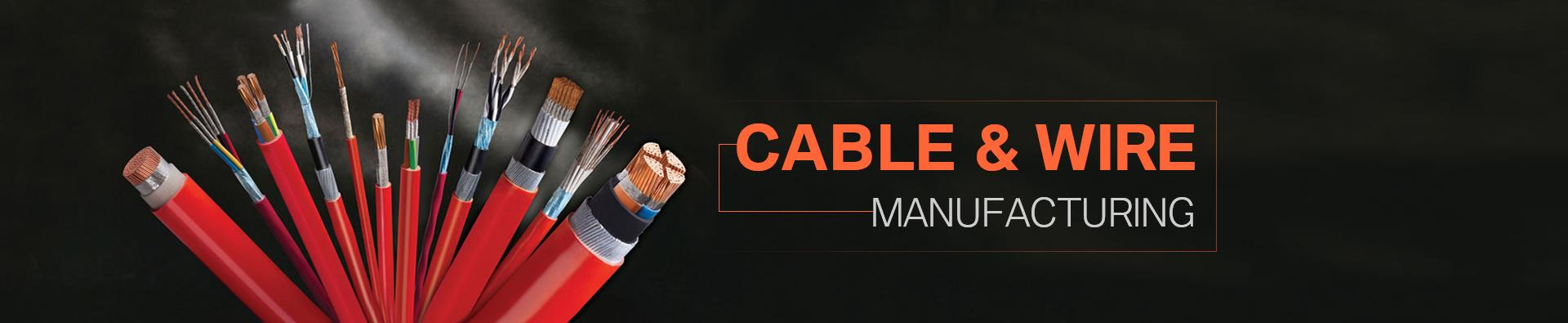Categories

Office Mob: +8615524105871

Office Tel: +86-024-31931990

Office Fax: +86-024-22845391

E-mail: info@eoexport.com

Knowledge
Home > Knowledge > Content
Wire and cable current carrying capacity calculation formula
May 18, 2018

Amount calculation formula

Estimates

Multiply the number by 9.5 and increase the number by 9.

Thirty-five to three-by-five, and both teams lose five points.

The conditions are changed and converted, and the high temperature 10% copper upgrade.

The number of pipes worn was 234, and the full load was 876.

Explanation: In this section, the current-carrying capacity (safety current) of various insulated wires (rubber and plastic insulated wire) is not directly indicated, but is expressed by “multiply the cross-section by a certain factor”, which is calculated by mental arithmetic. It can be seen from Table 53 that the multiple decreases with the increase of the cross section.

Multiply the number by two in five and increase the number by one.

“Five to multiply by two at five, and one at a time to go down” is to say that various sections of aluminum-core insulated wire with a diameter of 2.5 mm or less have a current carrying capacity of approximately 9 times the number of sections. With a 2.5mm' wire, the current carrying capacity is 2.5 x 9 = 22.5 (A). The multiple of the current-carrying capacity and the number of cross-sections of the conductors from 4 mm' and above is the upper row along the line number, and the multiple is successively reduced by 1 (4×8, 6×7, 10×6, 16×5, 25×4).

Thirty-five to three-by-five, and both teams lose five points.

"Thirty-five-by-three and five-points, both in groups minus five," said 35mm" wire carrying capacity is 3.5 times the number of sections, that is 35 × 3.5 = 122.5 (A). For a conductor of 50mm' or more, the multiple relationship between the current carrying capacity and the number of sections becomes a group of two two wire numbers, and the multiple is reduced by 0.5. That is, the current carrying capacity of the 50 and 70mm' wires is the number of sections. Times; 95,120mm" wire carrying capacity is 2.5 times its cross-sectional area, and so on.

The conditions are changed and converted, and the high temperature 10% copper upgrade.

"Conditions are subject to change, high temperature 10% copper upgrade". The above-mentioned mouthpiece is made of an aluminum core insulated wire and an open coating under the condition of an ambient temperature of 25°C. If the aluminum core insulation wire is deposited in an area where the ambient temperature is higher than 25°C for a long time, the wire carrying capacity can be calculated according to the above formula, and then it can be used for 10%. When the aluminum wire is used instead of the aluminum wire, the copper core wire is used. Its ampacity is slightly larger than that of the aluminum wire of the same specification. The ampacity method can be used to calculate the ampacity of a line number larger than that of the aluminum wire. For example, the current carrying capacity of 16mm' copper wire can be calculated by 25mm2 aluminum wire.

The number of pipes worn was 234, and the full load was 876.

"When the number of pipes is 234 and 876 is full, the current is carried by two pipes, three pipes, and four electric pipes. When the pipe is laid with two, three, or four wires, the current-carrying capacity is 80% of the electric load calculated by the electrician alone. , 70%, 60%.

• Categories